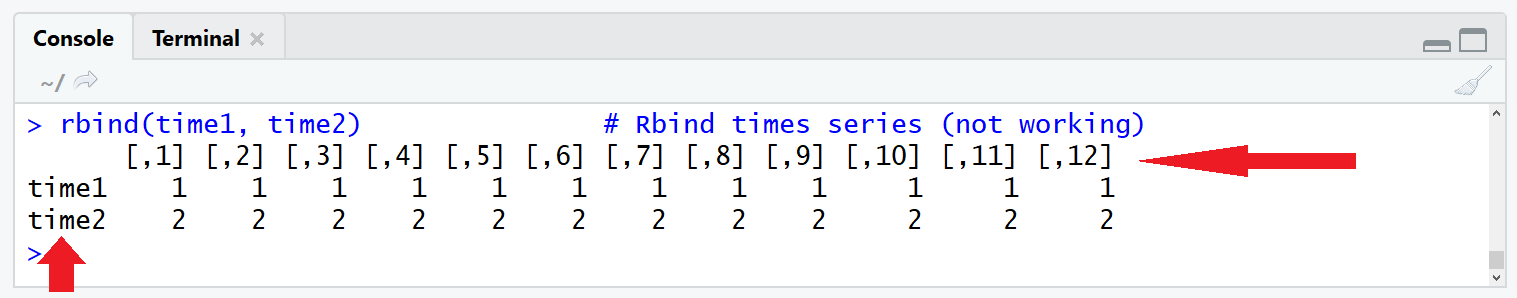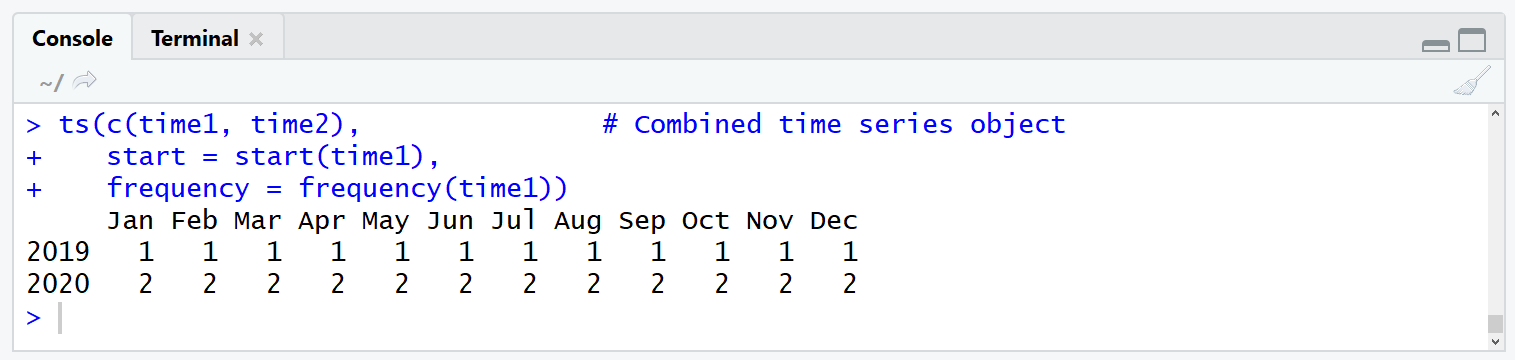# Merge Time Series in R (Example)

In this R tutorial, I’ll explain how to merge two time series objects in the R programming language.

The tutorial will contain the following contents:

Let’s dive into it!

## Example Data

Let’s create two time series objects (ts) in R that we can use in the example later on:

```time1 <- ts(rep(1, 12),           # Create first time series
start = c(2019, 1),
frequency = 12)
time2 <- ts(rep(2, 12),           # Create second time series
start = c(2020, 1),
frequency = 12)```

## Merge Time Series in R

Typically, we would merge two objects above each other with the rbind R function. Let’s see how this looks with ts objects:

`rbind(time1, time2)               # Rbind times series (not working)`Figure 1: Merging Time Series with rbind() is not Working Properly.

Not good! As Figure 1 shows, the times series attributes are lost, when we use the rbind function.

The correct way to combine multiple ts objects is the following:

```ts(c(time1, time2),               # Combined time series object
start = start(time1),
frequency = frequency(time1))```Figure 2: ts Function with Frequency Specification Works Well.

Figure 2 shows how a good merging of two time series objects should look like.

## Video & Further Resources on the Topic

Do you need further info on the R code of this article? Then you might have a look at the following video on my YouTube channel. In the video, I’m explaining the contents of this post.

Please accept YouTube cookies to play this video. By accepting you will be accessing content from YouTube, a service provided by an external third party.If you accept this notice, your choice will be saved and the page will refresh.

Have a look at the following video of Jordan Kern’s YouTube channel. In the video, he illustrates how to analyze time series data.

Please accept YouTube cookies to play this video. By accepting you will be accessing content from YouTube, a service provided by an external third party.If you accept this notice, your choice will be saved and the page will refresh.

Furthermore, you may have a look at some of the other articles on my homepage.

In this article, you learned how to retain the structure of time series data when it is combined in the R programming language. Don’t hesitate to let me know in the comments section, in case you have further questions.

Subscribe to the Statistics Globe Newsletter

•May 13, 2020 4:51 am

Hi, I want to know about start=. How to use the same start= in combine ? Because it changes to every year.

•May 13, 2020 5:48 am